×#### Thank you for registering.

One of our academic counsellors will contact you within 1 working day.

Click to Chat

1800-1023-196

+91-120-4616500

CART 0

• 0

MY CART (5)

Use Coupon: CART20 and get 20% off on all online Study Material

ITEM
DETAILS
MRP
DISCOUNT
FINAL PRICE
Total Price: Rs.

There are no items in this cart.
Continue Shopping• Complete JEE Main/Advanced Course and Test Series
• OFFERED PRICE: Rs. 15,900
• View Details

```Chapter 9: Ratio and Proportion Exercise – 9.1

Question: 1

If x: y = 3: 5, find the ratio 3x + 4y: 8x + 5y.

Solution: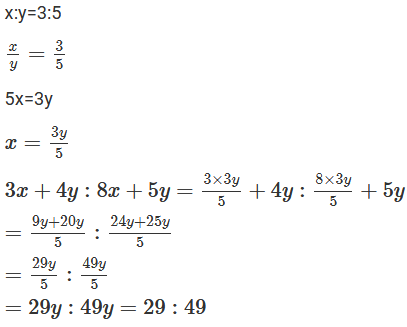Question: 2

If x: y = 8: 9, find the ratio (7x - 4y): 3x + 2y.

Solution:Question: 3

If two numbers are in the ratio 6:13 and their L.C.M is 312, find the numbers.

Solution: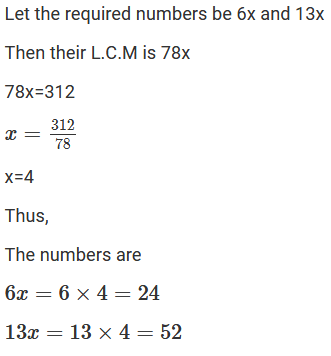Question: 4

Two numbers are in the ratio 3: 5. If 8 is added to each number, the ratio becomes 2: 3. Find the numbers

Solution:Question: 5

What should be added to each term of the ratio 7: 13 so that the ratio becomes 2: 3

Solution: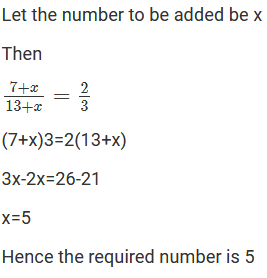Question: 6

Three numbers are in the ratio 2: 3: 5 and the sum of these numbers is 800. Find the numbers

Solution:Question: 7

The ages of two persons are in the ratio 5: 7. Eighteen years ago their ages were in the ratio 8: 13. Find their present ages.

Solution: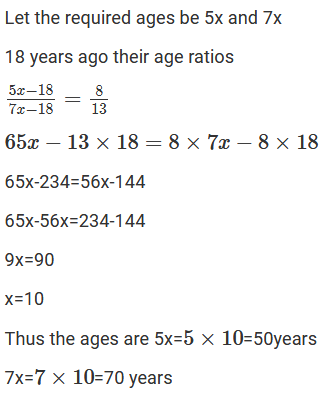Question: 8

Two numbers are in the ratio 7: 11. If 7 is added to each of the numbers, the ratio becomes 2: 3. Find the numbers.

Solution:Question: 9

Two numbers are in the ratio 2: 7. 11 the sum of the numbers is 810. Find the numbers.

Solution:Question: 10

Divide Rs 1350 between Ravish and Shikha in the ratio 2: 3.

Solution:Question: 11

Divide Rs 2000 among P, Q, R in the ratio 2: 3: 5.

Solution:Question: 12

The boys and the girls in a school are in the ratio 7:4. If total strength of the school be 550, find the number of boys and girls.

Solution:Question: 13

The ratio of monthly income to the savings of a family is 7: 2. If the savings be of Rs. 500, find the income and expenditure.

Solution:

It is given that

The ratio of income and savings is 7: 2

Savings

2x = 500

So, x = 250

Therefore,

Income = 7 × 250 = 1750

Expenditure = Income - savings

= 1750 - 500

= Rs.1250

Question: 14

The sides of a triangle are in the ratio 1: 2: 3. If the perimeter is 36 cm, find its sides.

Solution: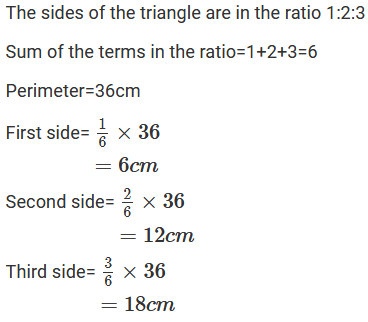Question: 15

A sum of Rs 5500 is to be divided between Raman and Amen in the rate 2: 3. How much will each get?

Solution: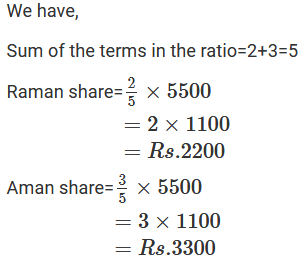Question: 16

The ratio of zinc and copper in an alloy is 7: 9. It the weight of the copper in the alloy is 11.7 kg, find the weight of the zinc in the alloy.

Solution: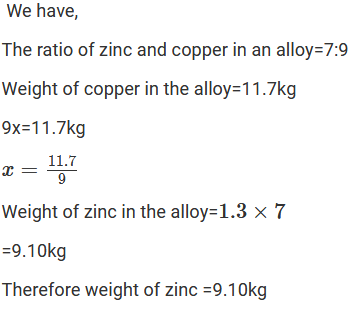Question: 17

In the ratio 7: 8. If the consequent is 40, what a the antecedent

Solution:

Given ratio = 7: 8

Consequent

8x = 40

x = 40/8

x = 5

antecedent = 7x = 7 × 5 = 35

Question: 18

Divide Rs 351 into two parts such that one may be to the other as 2: 7.

Solution:Question: 19

Find the ratio of the price of pencil to that of ball pen, if pencil cost Rs.16 per score and ball pen cost Rs.8.40 per dozen.

Solution:Question: 20

In a class, one out of every six students fails. If there are 42 students in the class, how many pass?

Solution:

Given,

One out of 6 student fails

x out of 42 students

16 = x/42

x = 42/6

x = 7

Number of students who fail = 7 students

No of students who pass =Total students - Number of students who fail = 42 – 7 = 35 students.
```### Course Features

• 728 Video Lectures
• Revision Notes
• Previous Year Papers
• Mind Map
• Study Planner
• NCERT Solutions
• Discussion Forum
• Test paper with Video Solution